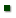Skip to main content
ARS Home » Research » Publications at this Location » Publication #146231

Title: A DYNAMIC MODEL TO PREDICT THE COMPOSITION OF FAT-FREE MATTER GAINS IN CATTLE

AuthorWilliams, Charles

 Submitted to: Journal of Animal Science Supplement Publication Type: Abstract Only Publication Acceptance Date: 3/10/2003 Publication Date: 7/1/2003 Citation: WILLIAMS, C.B. A DYNAMIC MODEL TO PREDICT THE COMPOSITION OF FAT-FREE MATTER GAINS IN CATTLE. JOURNAL OF ANIMAL SCIENCE. 2003. v. 81(SUPP.1): ABSTRACT P. 307. Interpretive Summary: Technical Abstract: Composition of empty BW (EBW) was described in terms of ether-extractable lipid (FAT) and fat-free matter(FFM) and the terms dEBW, dFAT, and dFFM were used to represent daily gains in these components. The dFFM is composed of protein, water, and ash, and a model was developed to predict the composition of dFFM. The conceptual approach used in model development was based on experimental data that showed as cattle grew from birth to maturity: a) the water content of FFM decreased and the protein and ash content increased, b) the protein content of FFM increased at a decreasing rate, and c) the protein:ash ratio in the FFM dry matter was fixed. These results suggest that as cattle grow and mature, gains in FFM would contain increasing amounts of protein, and the protein content of dFFM would increase at a decreasing rate as the dFFM content of dEBW decreased. Mathematical functions were formulated to represent these concepts, and a set of equations were derived to predict composition of dFFM. The protein content of dFFM was predicted as a function of the fraction of dEBW that was dFFM, FAT content of EBW, and dFFM. A fixed protein to ash ratio of 4.26:1 was used to calculate the amount of ash, and water was obtained as a residual. Gain in EBW, dFAT, and dFFM of Hereford x Angus steers from birth to 500 kg BW was simulated with a previously published model, and the above model was used to predict composition of dFFM. Predicted response curves of the EBW components over the growing period were similar in shape to observed data. Regression analysis was used to investigate the relationship between protein weight and FFM weight. Results showed a linear relationship with no evidence for curvilinearity in the predicted data and two experimental data sets. The coefficient on FFM in the predicted data was 0.249 (SE=0.0008), and in the two sets of experimental data, the coefficients on FFM were 0.247 (SE=0.003) and 0.25 (SE=0.004). These results support the conclusion that the model is capable of accurately representing the real system.